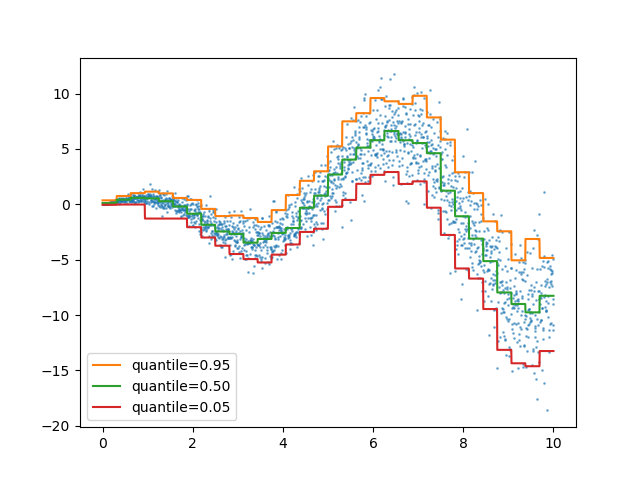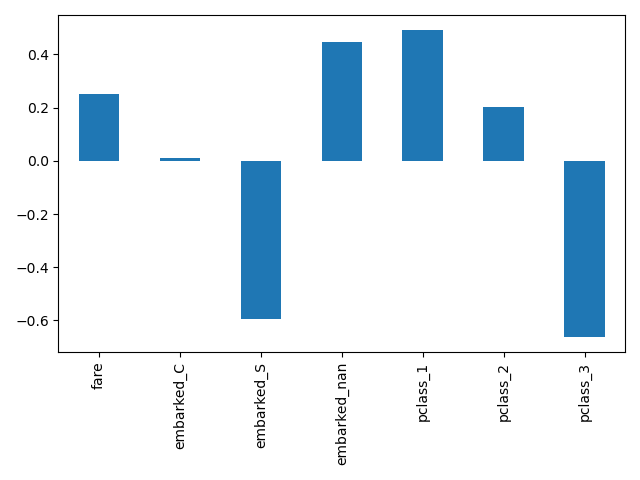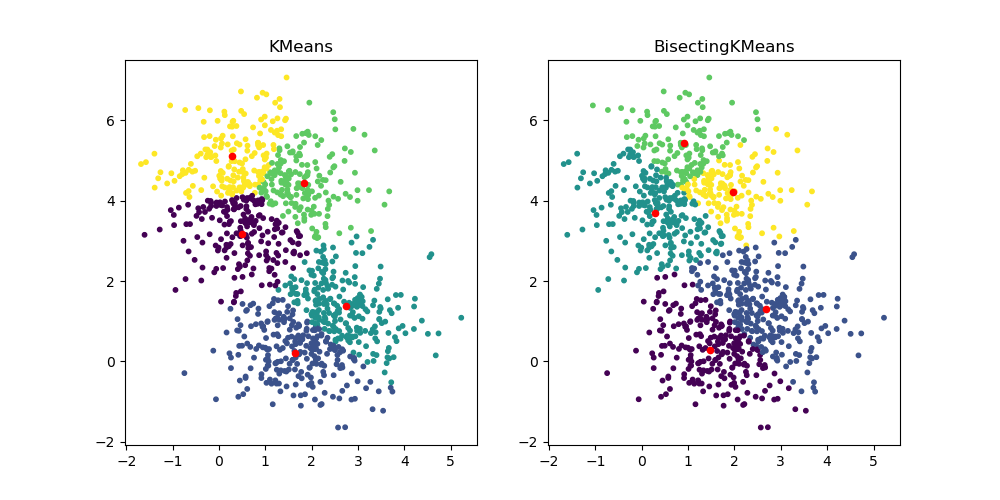# Release Highlights for scikit-learn 1.1¶

We are pleased to announce the release of scikit-learn 1.1! Many bug fixes and improvements were added, as well as some new key features. We detail below a few of the major features of this release. For an exhaustive list of all the changes, please refer to the release notes.

pip install --upgrade scikit-learn


or with conda:

conda install -c conda-forge scikit-learn


## Quantile loss in ensemble.HistGradientBoostingRegressor¶

ensemble.HistGradientBoostingRegressor can model quantiles with loss="quantile" and the new parameter quantile.

from sklearn.ensemble import HistGradientBoostingRegressor
import numpy as np
import matplotlib.pyplot as plt

# Simple regression function for X * cos(X)
rng = np.random.RandomState(42)
X_1d = np.linspace(0, 10, num=2000)
X = X_1d.reshape(-1, 1)
y = X_1d * np.cos(X_1d) + rng.normal(scale=X_1d / 3)

quantiles = [0.95, 0.5, 0.05]
parameters = dict(loss="quantile", max_bins=32, max_iter=50)
hist_quantiles = {
**parameters, quantile=quantile
).fit(X, y)
for quantile in quantiles
}

fig, ax = plt.subplots()
ax.plot(X_1d, y, "o", alpha=0.5, markersize=1)
for quantile, hist in hist_quantiles.items():
ax.plot(X_1d, hist.predict(X), label=quantile)
_ = ax.legend(loc="lower left")## get_feature_names_out Available in all Transformers¶

get_feature_names_out is now available in all Transformers. This enables pipeline.Pipeline to construct the output feature names for more complex pipelines:

from sklearn.compose import ColumnTransformer
from sklearn.preprocessing import OneHotEncoder, StandardScaler
from sklearn.pipeline import make_pipeline
from sklearn.impute import SimpleImputer
from sklearn.feature_selection import SelectKBest
from sklearn.datasets import fetch_openml
from sklearn.linear_model import LogisticRegression

X, y = fetch_openml("titanic", version=1, as_frame=True, return_X_y=True)
numeric_features = ["age", "fare"]
numeric_transformer = make_pipeline(SimpleImputer(strategy="median"), StandardScaler())
categorical_features = ["embarked", "pclass"]

preprocessor = ColumnTransformer(
[
("num", numeric_transformer, numeric_features),
(
"cat",
OneHotEncoder(handle_unknown="ignore", sparse=False),
categorical_features,
),
],
verbose_feature_names_out=False,
)
log_reg = make_pipeline(preprocessor, SelectKBest(k=7), LogisticRegression())
log_reg.fit(X, y)

Pipeline(steps=[('columntransformer',
ColumnTransformer(transformers=[('num',
Pipeline(steps=[('simpleimputer',
SimpleImputer(strategy='median')),
('standardscaler',
StandardScaler())]),
['age', 'fare']),
('cat',
OneHotEncoder(handle_unknown='ignore',
sparse=False),
['embarked', 'pclass'])],
verbose_feature_names_out=False)),
('selectkbest', SelectKBest(k=7)),
('logisticregression', LogisticRegression())])
In a Jupyter environment, please rerun this cell to show the HTML representation or trust the notebook.

Here we slice the pipeline to include all the steps but the last one. The output feature names of this pipeline slice are the features put into logistic regression. These names correspond directly to the coefficients in the logistic regression:

import pandas as pd

log_reg_input_features = log_reg[:-1].get_feature_names_out()
pd.Series(log_reg[-1].coef_.ravel(), index=log_reg_input_features).plot.bar()
plt.tight_layout()## Grouping infrequent categories in OneHotEncoder¶

OneHotEncoder supports aggregating infrequent categories into a single output for each feature. The parameters to enable the gathering of infrequent categories are min_frequency and max_categories. See the User Guide for more details.

from sklearn.preprocessing import OneHotEncoder
import numpy as np

X = np.array(
[["dog"] * 5 + ["cat"] * 20 + ["rabbit"] * 10 + ["snake"] * 3], dtype=object
).T
enc = OneHotEncoder(min_frequency=6, sparse=False).fit(X)
enc.infrequent_categories_

[array(['dog', 'snake'], dtype=object)]


Since dog and snake are infrequent categories, they are grouped together when transformed:

encoded = enc.transform(np.array([["dog"], ["snake"], ["cat"], ["rabbit"]]))
pd.DataFrame(encoded, columns=enc.get_feature_names_out())

x0_cat x0_rabbit x0_infrequent_sklearn
0 0.0 0.0 1.0
1 0.0 0.0 1.0
2 1.0 0.0 0.0
3 0.0 1.0 0.0

## Performance improvements¶

Reductions on pairwise distances for dense float64 datasets has been refactored to better take advantage of non-blocking thread parallelism. For example, neighbors.NearestNeighbors.kneighbors and neighbors.NearestNeighbors.radius_neighbors can respectively be up to ×20 and ×5 faster than previously. In summary, the following functions and estimators now benefit from improved performance:

To know more about the technical details of this work, you can read this suite of blog posts.

Moreover, the computation of loss functions has been refactored using Cython resulting in performance improvements for the following estimators:

## MiniBatchNMF: an online version of NMF¶

The new class decomposition.MiniBatchNMF implements a faster but less accurate version of non-negative matrix factorization (decomposition.NMF). MiniBatchNMF divides the data into mini-batches and optimizes the NMF model in an online manner by cycling over the mini-batches, making it better suited for large datasets. In particular, it implements partial_fit, which can be used for online learning when the data is not readily available from the start, or when the data does not fit into memory.

import numpy as np
from sklearn.decomposition import MiniBatchNMF

rng = np.random.RandomState(0)
n_samples, n_features, n_components = 10, 10, 5
true_W = rng.uniform(size=(n_samples, n_components))
true_H = rng.uniform(size=(n_components, n_features))
X = true_W @ true_H

nmf = MiniBatchNMF(n_components=n_components, random_state=0)

for _ in range(10):
nmf.partial_fit(X)

W = nmf.transform(X)
H = nmf.components_
X_reconstructed = W @ H

print(
f"relative reconstruction error: ",
f"{np.sum((X - X_reconstructed) ** 2) / np.sum(X**2):.5f}",
)

relative reconstruction error:  0.00364


## BisectingKMeans: divide and cluster¶

The new class cluster.BisectingKMeans is a variant of KMeans, using divisive hierarchical clustering. Instead of creating all centroids at once, centroids are picked progressively based on a previous clustering: a cluster is split into two new clusters repeatedly until the target number of clusters is reached, giving a hierarchical structure to the clustering.

from sklearn.datasets import make_blobs
from sklearn.cluster import KMeans, BisectingKMeans
import matplotlib.pyplot as plt

X, _ = make_blobs(n_samples=1000, centers=2, random_state=0)

km = KMeans(n_clusters=5, random_state=0).fit(X)
bisect_km = BisectingKMeans(n_clusters=5, random_state=0).fit(X)

fig, ax = plt.subplots(1, 2, figsize=(10, 5))
ax.scatter(X[:, 0], X[:, 1], s=10, c=km.labels_)
ax.scatter(km.cluster_centers_[:, 0], km.cluster_centers_[:, 1], s=20, c="r")
ax.set_title("KMeans")

ax.scatter(X[:, 0], X[:, 1], s=10, c=bisect_km.labels_)
ax.scatter(
bisect_km.cluster_centers_[:, 0], bisect_km.cluster_centers_[:, 1], s=20, c="r"
)
_ = ax.set_title("BisectingKMeans")Total running time of the script: ( 0 minutes 9.112 seconds)

Gallery generated by Sphinx-Gallery